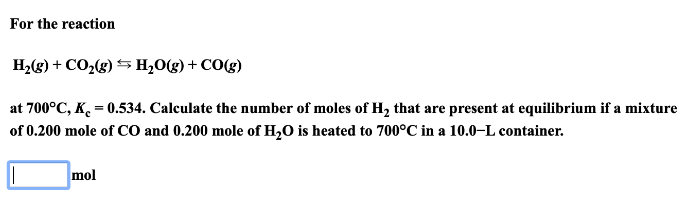# For the reaction H2(g) + CO2(g) ⇌ H2O(g) + CO(g) at 700 degrees C, Kc= 0.534. Calculate the number of moles of H2 that are present at equilibrium if a mixture of 0.200 mole of CO and 0.200 mole of H2O is heated to 700 degrees C in a 10.0-L container.